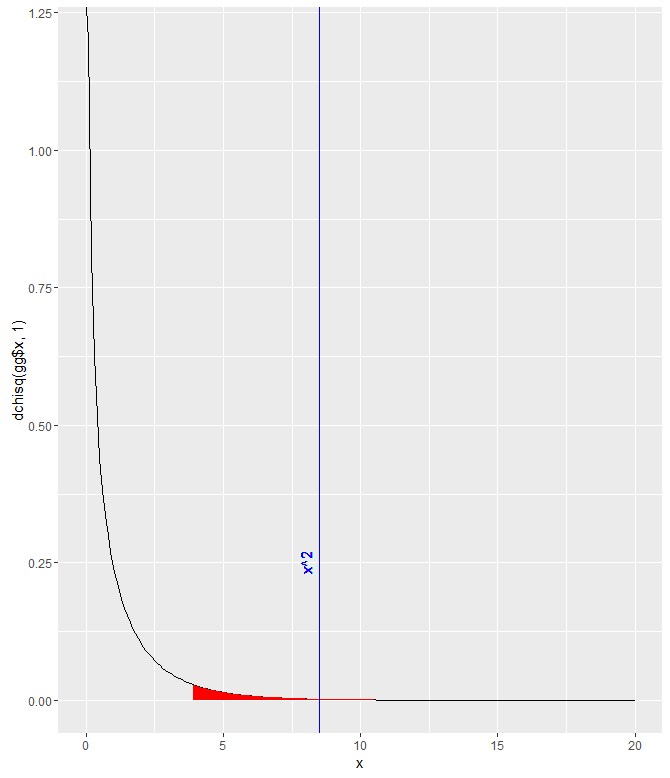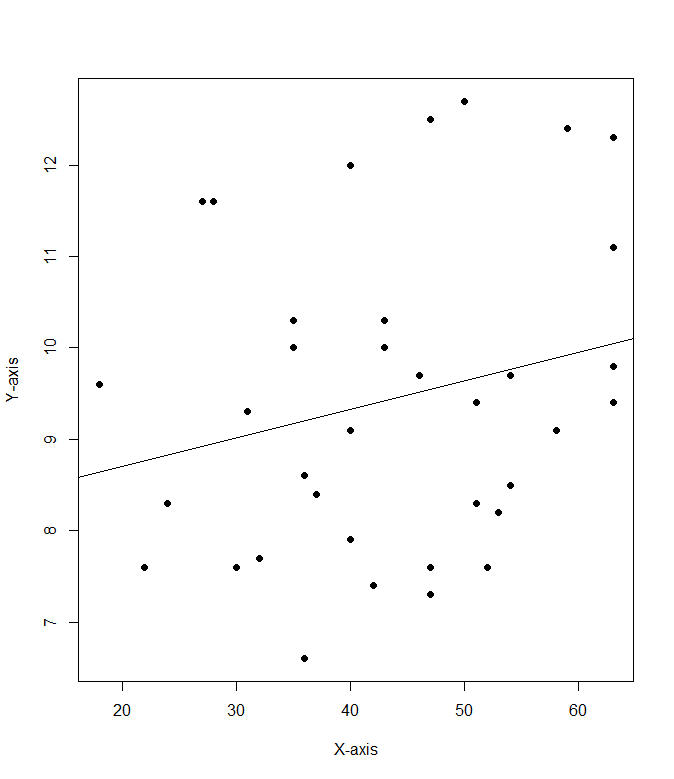Select Page

Chi-Squared testing

Null hypothesis:  The proportion of people who choose the same hotel again will be the same for beach combers and windsurfers.

To start off, I constructed my data.frame in a very roundabout manner:

>Beachcomber <- c(163,64,227)
>Windsurfer <- c(154,108,262)
>Choose_again <- c("Yes", "No", "Total")
>dat <- data.frame(Choose_again, Beachcomber, Windsurfer)
>dat\$Total <- dat\$Beachcomber + dat\$Windsurfer
>dat <- dat[,-1]
>rownames(dat) <- c("Yes", "no","total")

>dat

Beachcomber Windsurfer Total
Yes           163        154   317
no             64        108   172
total         227        262   489

Next, I run the chi-test and save it in an object:

>res<-chisq.test(dat[1:2,1:2])
> res

Pearson's Chi-squared test with Yates' continuity correction

data: dat[1:2, 1:2]
X-squared = 8.4903, df = 1, p-value = 0.0035

I was stuck on that for a while, trying to figure out exactly which parts of the data.frame needed to be included/excluded.  I did notice that this way of doing it didn’t give me the error that other combinations did though.  I assume it’s because the function implemented Yates’ continuity correction, which is automatically implemented for 2×2 tables.  The p-value is quite small, so at a threshold of p=.05, the null hypothesis would be rejected and we’d say that it appears that the proportion of people who choose to return to their hotel is different for each group.gg <- data.frame(x = seq(0,20,.1))
gg\$y <- dchisq(gg\$x, 1)
ggplot(gg) +
geom_path(aes(x,y)) +
geom_ribbon(data=gg[gg\$x>qchisq(.05,1,lower.tail=FALSE),], aes(x,ymin=0, ymax=y), fill="red")+
geom_vline(xintercept = res\$statistic, color = "blue")+
labs( x = "x", y = "dchisq(gg\$x, 1)")+
geom_text(aes(x=8, label="x^2", y=0.25), colour="blue", angle=90)

ANOVA

> high <- c(10,9,8,9,10,8))
> moderate <- c(8,10,6,7,8,8)
> low <- c(4,6,6,4,2,2)
> reaction <- c(10,9,8,9,10,8,8,10,6,7,8,8,4,6,6,4,2,2)
> stresslvls <- c(rep("high",6), rep("moderate",6), rep("low",6))
> dat <- data.frame(reaction,stresslvls)
> analysis <- lm(reaction ~ as.factor(stresslvls), data = dat)
> anova(analysis)
Analysis of Variance Table

Response: stress
Df Sum Sq Mean Sq  F value     Pr(>F)
as.factor(stresslvls)  2 82.111  41.056   21.358  4.082e-05 ***
Residuals             15 28.833   1.922
---
Signif. codes: 0 ‘***’ 0.001 ‘**’ 0.01 ‘*’ 0.05 ‘.’ 0.1 ‘ ’ 1

The ANOVA test essentially breaks a sample down into smaller groups and compares the variance between the means of each of those groups to determine how much variance can be attributed to chance.

Here, we have analyzed a grouping of the reaction times of participants that were subjected to various levels of stress.  Above, we can see the return of the ANOVA test.  We can see that the between-group mean sum of squares is 41.056.  This shows that the difference between each of the individual sample means and the mean of all the samples.  The F value is the ratio between the mean squares of the mean squares.  The larger the F statistic is, the more you can rule out the difference between the means being due to chance.  The fact that the P value is so small allows us to reject the null hypothesis and claim that the level of stress the participants are subjected does have an effect on their reaction times.

T-test for Independent Means

1. The mean for males is 3.571429 and the mean for females is 7.6
2. The degrees of freedom for the t.test is 6.7687
3. The T statistic is 2.8651
4. The P value is 0.02505
5. Yes
6. +- 2.576

Correlation & Regression

1a.  H0: A cookie will break at more than 70 pounds of force or greater; u >= 70

Ha: A cookie will break at less than 70 pounds of force on average; u < 70

1b.  yes

1c.   p = 0.0359  There’s a 3.59% chance of finding a sample mean of 69.1 if u >=70.

1d.  p = 0.0002  since p < 0.5, there is enough evidence to dismiss H0

1e.  p = 0.0227  since p < 0.5, there is enough evidence to dismiss H0

2a.  cor(mod8\$Cost.per.serving, mod8\$Fibber.per.serving)

2b.  .228

2c:Q1.  I created a function to do the calculations to find mu for the Confidence Interval:

ci <- function(xbar, con, sig, n)
{
con1 = con + ((1-con)/2)
mu = xbar + (qnorm(con1) * (sig / sqrt(n)))
sn = xbar – (qnorm(con1) * (sig / sqrt(n)))
paste(“The confidence interval is between “, sn, ” and “, +mu, ” and the estimated mean is “, xbar, sep =””)
}

The confidence intervals came in as follows:

> ci(115, .9, 15, 100)
 “The confidence interval is between 112.532719559573 and 117.467280440427 and the estimated mean is 115”
> ci(115, .95, 15, 100)
 “The confidence interval is between 112.06005402319 and 117.93994597681 and the estimated mean is 115”
> ci(115, .98, 15, 100)
 “The confidence interval is between 111.510478188939 and 118.489521811061 and the estimated mean is 115”

Q2

 “The confidence interval is between 83.0400360154599 and 86.9599639845401 and the estimated mean is 85”

Q3

((1.96 * 15) / 5)^2
 34.5744

I think the minimum sample size should be 35 if I’m thinking of the zscore tails properly.

edit: After reviewing the last question, 35 is right, but how i got to it was wrong

III

The biggest thing to note about this is the difference between the 95% confidence interval and the 97.5th percentile ranking.  Each tail of the 95% confidence interval contains 2.5% of the area under the curve.  But we’re only looking for a single tailed value, so we must use qnorm(.975).

I feel like I was perhaps a little bit confused about what questions 1 and 2 were asking for, or what the answer should look like.